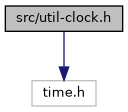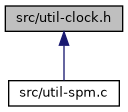suricata
util-clock.h File Reference
`#include <time.h>`
Include dependency graph for util-clock.h:This graph shows which files directly or indirectly include this file:Go to the source code of this file.

## Macros

#define CLOCK_INIT   clock_t clo1, clo2; clo1 = clo2 = 0;

#define CLOCK_START   clo1 = clock()

#define CLOCK_END   clo2 = clock()

#define CLOCK_PRINT_SEC   printf("Seconds spent: %.4fs\n", ((clo2 - clo1)/(double)CLOCKS_PER_SEC))

#define GET_CLOCK_END_SECS   ((clo1 - clo2)/(double)CLOCKS_PER_SEC)

## Detailed Description

Definition in file util-clock.h.

## ◆ CLOCK_END

 #define CLOCK_END   clo2 = clock()

Definition at line 35 of file util-clock.h.

## ◆ CLOCK_INIT

 #define CLOCK_INIT   clock_t clo1, clo2; clo1 = clo2 = 0;

Definition at line 32 of file util-clock.h.

## ◆ CLOCK_PRINT_SEC

 #define CLOCK_PRINT_SEC   printf("Seconds spent: %.4fs\n", ((clo2 - clo1)/(double)CLOCKS_PER_SEC))

Definition at line 37 of file util-clock.h.

## ◆ CLOCK_START

 #define CLOCK_START   clo1 = clock()

Definition at line 33 of file util-clock.h.

## ◆ GET_CLOCK_END_SECS

 #define GET_CLOCK_END_SECS   ((clo1 - clo2)/(double)CLOCKS_PER_SEC)

Definition at line 39 of file util-clock.h.